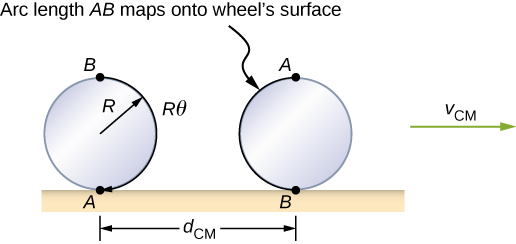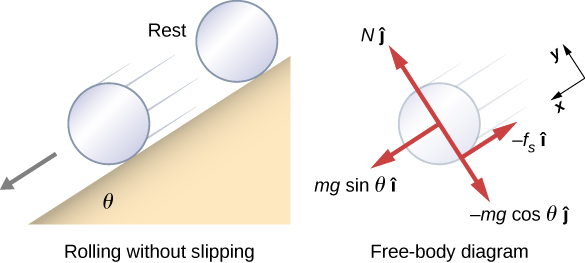# 11.1 Rolling motion  (Page 2/6)

 Page 2 / 6

From [link] (a), we see the force vectors involved in preventing the wheel from slipping. In (b), point P that touches the surface is at rest relative to the surface. Relative to the center of mass, point P has velocity $\text{−}R\omega \stackrel{^}{i}$ , where R is the radius of the wheel and $\omega$ is the wheel’s angular velocity about its axis. Since the wheel is rolling, the velocity of P with respect to the surface is its velocity with respect to the center of mass plus the velocity of the center of mass with respect to the surface:

${\stackrel{\to }{v}}_{P}=\text{−}R\omega \stackrel{^}{i}+{v}_{\text{CM}}\stackrel{^}{i}.$

Since the velocity of P relative to the surface is zero, ${v}_{P}=0$ , this says that

${v}_{\text{CM}}=R\omega .$

Thus, the velocity of the wheel’s center of mass is its radius times the angular velocity about its axis. We show the correspondence of the linear variable on the left side of the equation with the angular variable on the right side of the equation. This is done below for the linear acceleration.

If we differentiate [link] on the left side of the equation, we obtain an expression for the linear acceleration of the center of mass. On the right side of the equation, R is a constant and since $\alpha =\frac{d\omega }{dt},$ we have

${a}_{\text{CM}}=R\alpha .$

Furthermore, we can find the distance the wheel travels in terms of angular variables by referring to [link] . As the wheel rolls from point A to point B , its outer surface maps onto the ground by exactly the distance travelled, which is ${d}_{\text{CM}}.$ We see from [link] that the length of the outer surface that maps onto the ground is the arc length $R\theta \text{​}$ . Equating the two distances, we obtain

${d}_{\text{CM}}=R\theta .$As the wheel rolls on the surface, the arc length R θ from A to B maps onto the surface, corresponding to the distance d CM that the center of mass has moved.

## Rolling down an inclined plane

A solid cylinder rolls down an inclined plane without slipping, starting from rest. It has mass m and radius r . (a) What is its acceleration? (b) What condition must the coefficient of static friction ${\mu }_{\text{S}}$ satisfy so the cylinder does not slip?

## Strategy

Draw a sketch and free-body diagram, and choose a coordinate system. We put x in the direction down the plane and y upward perpendicular to the plane. Identify the forces involved. These are the normal force, the force of gravity, and the force due to friction. Write down Newton’s laws in the x - and y -directions, and Newton’s law for rotation, and then solve for the acceleration and force due to friction.

## Solution

1. The free-body diagram and sketch are shown in [link] , including the normal force, components of the weight, and the static friction force. There is barely enough friction to keep the cylinder rolling without slipping. Since there is no slipping, the magnitude of the friction force is less than or equal to ${\mu }_{S}N$ . Writing down Newton’s laws in the x - and y -directions, we have
$\sum {F}_{x}=m{a}_{x};\phantom{\rule{0.5em}{0ex}}\sum {F}_{y}=m{a}_{y}.$A solid cylinder rolls down an inclined plane without slipping from rest. The coordinate system has x in the direction down the inclined plane and y perpendicular to the plane. The free-body diagram is shown with the normal force, the static friction force, and the components of the weight m g → . Friction makes the cylinder roll down the plane rather than slip.
Substituting in from the free-body diagram,
$\begin{array}{ccc}\hfill mg\phantom{\rule{0.2em}{0ex}}\text{sin}\phantom{\rule{0.2em}{0ex}}\theta -{f}_{\text{S}}& =\hfill & m{\left({a}_{\text{CM}}\right)}_{x},\hfill \\ \hfill N-mg\phantom{\rule{0.2em}{0ex}}\text{cos}\phantom{\rule{0.2em}{0ex}}\theta & =\hfill & 0,\hfill \\ \hfill {f}_{\text{S}}& \le \hfill & {\mu }_{\text{S}}N,\hfill \end{array}$
we can then solve for the linear acceleration of the center of mass from these equations:
${\left({a}_{\text{CM}}\right)}_{x}=g\left(\text{sin}\phantom{\rule{0.2em}{0ex}}\theta -{\mu }_{S}\text{cos}\phantom{\rule{0.2em}{0ex}}\theta \right).$

However, it is useful to express the linear acceleration in terms of the moment of inertia. For this, we write down Newton’s second law for rotation,
$\sum {\tau }_{\text{CM}}={I}_{\text{CM}}\alpha .$

The torques are calculated about the axis through the center of mass of the cylinder. The only nonzero torque is provided by the friction force. We have
${f}_{\text{S}}r={I}_{\text{CM}}\alpha .$

Finally, the linear acceleration is related to the angular acceleration by
${\left({a}_{\text{CM}}\right)}_{x}=r\alpha .$

These equations can be used to solve for ${a}_{\text{CM}},\alpha ,\phantom{\rule{0.2em}{0ex}}\text{and}\phantom{\rule{0.2em}{0ex}}{f}_{\text{S}}$ in terms of the moment of inertia, where we have dropped the x -subscript. We write ${a}_{\text{CM}}$ in terms of the vertical component of gravity and the friction force, and make the following substitutions.
${a}_{\text{CM}}=g\text{sin}\phantom{\rule{0.2em}{0ex}}\theta -\frac{{f}_{\text{S}}}{m}$

${f}_{\text{S}}=\frac{{I}_{\text{CM}}\alpha }{r}=\frac{{I}_{\text{CM}}{a}_{\text{CM}}}{{r}^{2}}$
From this we obtain
$\begin{array}{cc}\hfill {a}_{\text{CM}}& =g\phantom{\rule{0.2em}{0ex}}\text{sin}\phantom{\rule{0.2em}{0ex}}\theta -\frac{{I}_{\text{CM}}{a}_{\text{CM}}}{m{r}^{2}},\hfill \\ & =\frac{mg\phantom{\rule{0.2em}{0ex}}\text{sin}\phantom{\rule{0.2em}{0ex}}\theta }{m+\left({I}_{\text{CM}}\text{/}{r}^{2}\right)}.\hfill \end{array}$

Note that this result is independent of the coefficient of static friction, ${\mu }_{\text{S}}$ .
Since we have a solid cylinder, from [link] , we have ${I}_{\text{CM}}=m{r}^{2}\text{/}2$ and
${a}_{\text{CM}}=\frac{mg\phantom{\rule{0.2em}{0ex}}\text{sin}\phantom{\rule{0.2em}{0ex}}\theta }{m+\left(m{r}^{2}\text{/}2{r}^{2}\right)}=\frac{2}{3}g\phantom{\rule{0.2em}{0ex}}\text{sin}\phantom{\rule{0.2em}{0ex}}\theta .$

Therefore, we have
$\alpha =\frac{{a}_{\text{CM}}}{r}=\frac{2}{3r}g\phantom{\rule{0.2em}{0ex}}\text{sin}\phantom{\rule{0.2em}{0ex}}\theta .$
2. Because slipping does not occur, ${f}_{\text{S}}\le {\mu }_{\text{S}}N$ . Solving for the friction force,
${f}_{\text{S}}={I}_{\text{CM}}\frac{\alpha }{r}={I}_{\text{CM}}\frac{\left({a}_{\text{CM}}\right)}{{r}^{2}}=\frac{{I}_{\text{CM}}}{{r}^{2}}\left(\frac{mg\phantom{\rule{0.2em}{0ex}}\text{sin}\phantom{\rule{0.2em}{0ex}}\theta }{m+\left({I}_{\text{CM}}\text{/}{r}^{2}\right)}\right)=\frac{mg{I}_{\text{CM}}\phantom{\rule{0.2em}{0ex}}\text{sin}\phantom{\rule{0.2em}{0ex}}\theta }{m{r}^{2}+{I}_{\text{CM}}}.$

Substituting this expression into the condition for no slipping, and noting that $N=mg\phantom{\rule{0.2em}{0ex}}\text{cos}\phantom{\rule{0.2em}{0ex}}\theta$ , we have
$\frac{mg{I}_{\text{CM}}\text{sin}\phantom{\rule{0.2em}{0ex}}\theta }{m{r}^{2}+{I}_{\text{CM}}}\le {\mu }_{\text{S}}mg\phantom{\rule{0.2em}{0ex}}\text{cos}\phantom{\rule{0.2em}{0ex}}\theta$

or
${\mu }_{\text{S}}\ge \frac{\text{tan}\phantom{\rule{0.2em}{0ex}}\theta }{1+\left(m{r}^{2}\text{/}{I}_{\text{CM}}\right)}.$

For the solid cylinder, this becomes
${\mu }_{\text{S}}\ge \frac{\text{tan}\phantom{\rule{0.2em}{0ex}}\theta }{1+\left(2m{r}^{2}\text{/}m{r}^{2}\right)}=\frac{1}{3}\text{tan}\phantom{\rule{0.2em}{0ex}}\theta .$

state the properties of a base
state 3 properties of a base
State three properties of a base
mubarak
a stone is projected from the top of the tower 24.5m high with velocity of 39.2m/s at an angle of 30° with horizontal compute time taken to hit he ground
it took a car driver 30mins to travel a distance of 50km.what is the average speed
Suppose the master cylinder in a hydraulic system is at a greater height than the cylinder it is controlling. Explain how this will affect the force produced at the cylinder that is being controlled.
Why is popo less than atmospheric? Why is popo greater than pipi?
Louise
The old rubber boot shown below has two leaks. To what maximum height can the water squirt from Leak 1? How does the velocity of water emerging from Leak 2 differ from that of Leak 1? Explain your responses in terms of energy.
Louise
David rolled down the window on his car while driving on the freeway. An empty plastic bag on the floor promptly flew out the window. Explain why.
the pressure differential exerted a force on the bag greater than the gravitational force holding it on the floor.
gtitboi
what is angular velocity
The rate of change in angular displacement is defined as angular velocity.
Manorama
a length of copper wire was measured to be 50m with an uncertainty of 1cm, the thickness of the wire was measured to be 1mm with an uncertainty of 0.01mm, using a micrometer screw gauge, calculate the of copper wire used
Mustapha
it took a car driver 30 mins to travel a distance of 50km what is the average speed .
Isiguzo
If centripetal force is directed towards the center,why do you feel that you're thrown away from the center as a car goes around a curve? Explain
if there is a centripetal force it means that there's also a centripetal acceleration, getting back to your question, just imagine what happens if you pull out of a car when it's quickly moving or when you try to stop when you are running fast, anyway, we notice that there's always a certain force..
Lindomar
... that tends to fight for its previous direction when you try to attribute to it an opposite one ou try to stop it.The same thing also happens whe a car goes around a curve, the car it self is designed to a"straight line"(look at the position of its tyres, mainly the back side ones), so...
Lindomar
... whenever it goes around a curve, it tends to throw away its the occupiers, it's given to the fact that it must interrupt its initial direction and take a new one.
Lindomar
Which kind of wave does wind form
calculate the distance you will travel if you mantain an average speed of 10N m/s for 40 second
400m/s
Feng
hw to calculate the momentum of the 2000.0 elephant change hunter at a speed of 7.50 m/s
how many cm makes 1 inches
2.5
omwoyo
2.54cm=1inche
omwoyo
how do we convert from m/s to km/hr
When paddling a canoe upstream, it is wisest to travel as near to the shore as possible. When canoeing downstream, it may be best to stay near the middle. Explain why?
Explain why polarization does not occur in sound
one ship sailing east with a speed of 7.5m/s passes a certain point at 8am and a second ship sailing north at the same speed passed the same point at 9.30am at what distance are they closet together and what is the distance between them then

#### Get Jobilize Job Search Mobile App in your pocket Now!ByBy Stephen VoronBy David GeltnerBy OpenStaxBy Richley CrapoBy Tony PizurBy Janet ForresterBy Richley CrapoBy OpenStaxBy Dionne Mahaffey